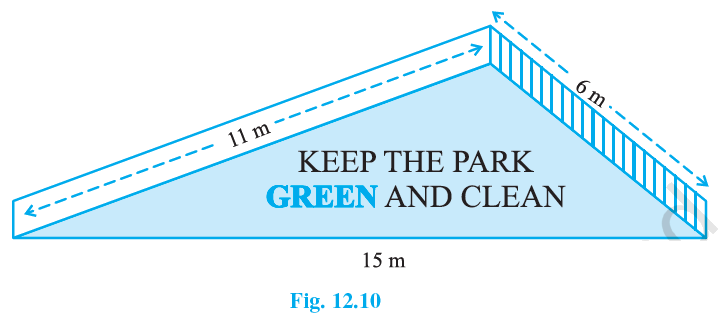# Ex.12.1 Q3 Heron’s Formula Solution - NCERT Maths Class 9

## Question

There is a slide in a park. One of its side walls has been painted in some colour with a message “KEEP THE PARK GREEN AND CLEAN” see Figure.  If the sides of the wall are $$15$$m, $$11$$m and $$6$$m, find the area painted in colour.## Text Solution

What is known?

Sides of the wall i.e. Dimensions of the triangle.

What is unknown?

Area of the (triangle) i.e. area of slope painted in colour.

Reasoning:

By using Heron’s formula we can calculate the area of triangle.

The formula given by Heron about the area of a triangle

$$=\sqrt{s(s-a)(s-b)(s-c)}$$

Where $$a, b$$ and $$c$$ are the sides of the triangle, and

\begin{align}s &= \text{Semi-perimeter}\\& = \begin{Bmatrix} \text{Half the Perimeter } \\ \text{ of the triangle} \;\end{Bmatrix} \\&=\frac{(a+b+c)}{2}\end{align}

Steps:

The sides of the walls (triangle) are $$a =11\; {\rm{m}} , b = 6 \, {\rm{m}}\;\text{and} \; c=15\, {\rm{m}}.$$

Semi Perimeter:

\begin{align}s & =\frac{(a+b+c)}{2} \\ & =\frac{11+6+15}{2} \\ &=\frac{32}{2} \\ &=16 \mathrm{m}\end{align}

By using Heron’s formula,

Area of a triangle

$$=\sqrt{s(s-a)(s-b)(s-c)}$$

Area of a triangle wall:

\begin{align}&=\sqrt{s(s-a)(s-b)(s-c)} \\ &=\!\sqrt{\!16(16\!-\!11)(16\!-\!6) (16\!-\!15) } \\ &=\sqrt{16 \times 5 \times 10 \times 1} \\ &=\sqrt{800} \mathrm{m}^{2} \\ &=20 \sqrt{2} \mathrm{m}^{2}\end{align}

Area of the wall of park painted in color $$= 20 \sqrt{2} \;\rm{m^2}$$

Learn from the best math teachers and top your exams

• Live one on one classroom and doubt clearing
• Practice worksheets in and after class for conceptual clarity
• Personalized curriculum to keep up with school